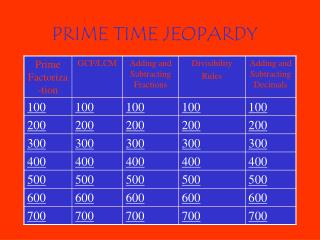DownloadDownload PresentationPRIME TIME JEOPARDY

# PRIME TIME JEOPARDY

Download Presentation## PRIME TIME JEOPARDY

- - - - - - - - - - - - - - - - - - - - - - - - - - - E N D - - - - - - - - - - - - - - - - - - - - - - - - - - -
##### Presentation Transcript

1. PRIME TIME JEOPARDY

2. Divisibility Rules for 700 Circle the numbers that are factors of the first number. 465 2 3 4 5 6 9 10 • Back

3. Divisibility Rules for 600 Circle the numbers that are factors of the first number. 562 2 3 4 5 6 9 10 • Back

4. Divisibility Rules for 500 Circle the numbers that are factors of the first number. 200 2 3 4 5 6 9 10 • Back

5. Divisibility Rules for 400 Circle the numbers that are factors of the first number. 78 2 3 4 5 6 9 10 Back

6. Divisibility Rules for 300 What is the divisibility rule for 9? Back

7. Divisibility Rules for 200 What is the divisibility rule for 6? • Back

8. Divisibility Rules for 100 Circle the numbers that are factors of the first number. 32 2 3 4 5 6 9 10 • Back

9. Prime Factorization for 700 Write the prime factorization using exponents. 200 • Back

10. Prime Factorization for 600 Write the prime factorization using exponents. 140 • Back

11. Prime Factorization for 500 Write the prime factorization using exponents. 85 • Back

12. Prime Factorization for 300 Write the prime factorization using exponents. 360 • Back

13. Prime Factorization for 200 Write the prime factorization using exponents. 60 Back

14. Prime Factorization for 400 Write the prime factorization using exponents. 155 Back

15. Prime Factorization for 100 Write the prime factorization using exponents. 80 • BACK

16. GCF/LCM for 100 Find the GCF/LCM for the following numbers 120 80 • Back

17. GCF/LCM for 200 Find the GCF/LCM for the following numbers 100 90 Back

18. GCF/LCM for 300 Find the GCF/LCM for the following numbers 240 180 • Back

19. GCF/LCM for 400 Find the GCF/LCM for the following numbers 250 225 • Back

20. GCF/LCM for 500 Find the GCF/LCM for the following numbers 300 275 • Back

21. GCF/LCM for 600 Find the GCF/LCM for the following numbers 180 60 • Back

22. GCF/LCM for 700 Find the GCF/LCM for the following numbers 420 200 • Back

23. Adding and Subtracting Fractions for 100 • 5/6 – 3/5 = ____ • Back

24. Adding and Subtracting Fractions for 200 1 and ¼ + 9 and ¾ = ____ Back

25. Adding and Subtracting Fractions for 300 2 and 3/5 + 8 and 6/7 = ____ • Back

26. Adding and Subtracting Fractions for 400 8/9 – 2/5 = ____ • Back

27. Adding and Subtracting Fractions for 500 13 and 4/5 + 17 and 3/8 = ___ • Back

28. Adding and Subtracting Fractions for 600 23 and 2/9 – 13 and 6/7 = ____ • Back

29. Adding and Subtracting Fractions for 700 15 and 1/8 – 13 and 3/5 = ____ • Back

30. Adding and Subtracting Decimals for 700 0.0865 + 3.2 + 45.66 - 12.84 = _____ • Back

31. Adding and Subtracting Decimals for 600 0.9 + 0.887 - .522 = ____ • Back

32. Adding and Subtracting Decimals for 500 56.7 – 9.76 – 1.235 = ____ • Back

33. Adding and Subtracting Decimals for 400 298.445 - 0.156 = _____ • Back

34. Adding and Subtracting Decimals for 300 5.6 + 9.887 + 0.061 = ____ • Back

35. Adding and Subtracting Decimals for 200 1.224 + 4.5 + 8.08 = ___ • Back

36. Adding and Subtracting Decimals for 100 0.525 + 0.1345 =___ Back